## Other Possible Shapes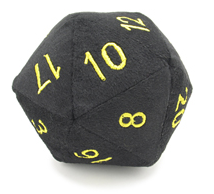One possible shape is a twenty-sided die, known as an icosahdron. The formula for its volume is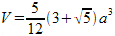. After substituting 500 for V, a is found to be approximately

6.1196

. This is then substituted into the formula for surface area,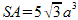. This yields a result of

324.3222 cm2

.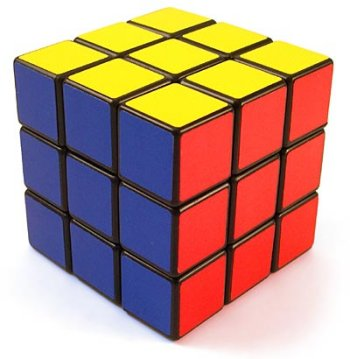Another alternative is a cube. Its volume is defined as

V=s3

. When solved with V=500, s comes to

7.937 cm

. When it is plugged into the formula

SA=6s2

, the surface area comes to

378 cm3

.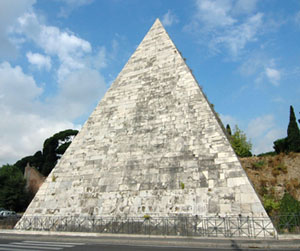If you were hoping for a more unique and distinguishable container, a pyramid or a cone would be a good choice. For both of these options, the simple formula for volume is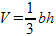. In solving for a square pyramid, it makes the most sense to leave b as the area of the entire base. Also, height must be rewritten in terms of b, which is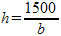. Since the surface area requires the slant height to determine the area of the triangles, that also needs to be found. The final equation is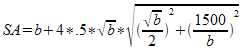. The graph of this looks like this: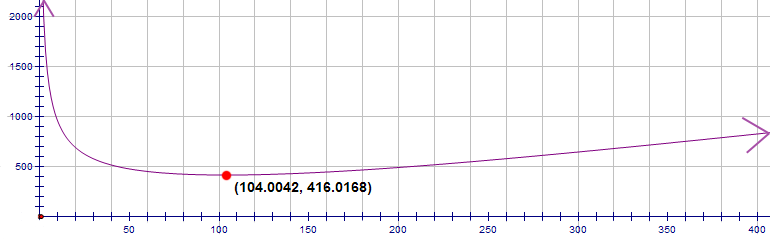The optimal point has a base area of

104.0042 cm2

, therefore a base side length of

10.1982 cm

, a height of

14.4225 cm

, and a surface area of

416.0168 cm2

.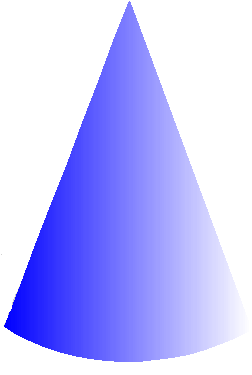With a cone, it makes more sense to use

pi*r2

for b. This gives a height of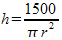and a surface area equation of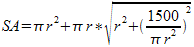with the graph: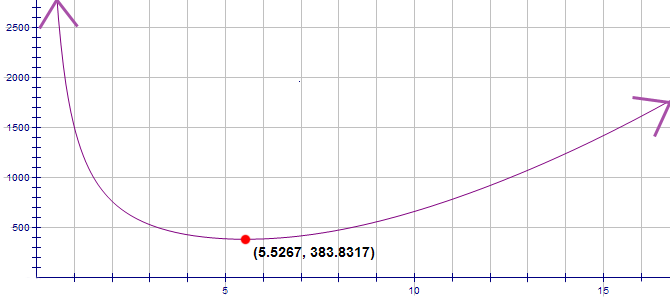At its optimal point, the cone has a base radius of

5.5267 cm

, a height of

15.6318 cm

, and a surface area of

383.8317 cm2

.